# Finding Roots of Quadratic Equation

### Finding Roots of Quadratic Equation

This trick will show how to define a customized function in Excel using Visual Basic for Applications (VBA). It covers in defining variables and creating simple mathematical expression in VBA. This particular example is about getting the roots of quadratic equation. It might sound difficult but it is pretty easy in solving inside Excel module.

Description of Example
The main objective of this example is to find the roots of quadratic equation. There is a known formula to solve the roots when the form of equation is ax2 + bx + c = 0. The generic formula are x1 = [-b + (b2 - 4ac)0.5] / 2a and x2 = [-b - (b2 - 4ac)0.5] / 2a. Where x1 and x2 are the roots of quadratic equation.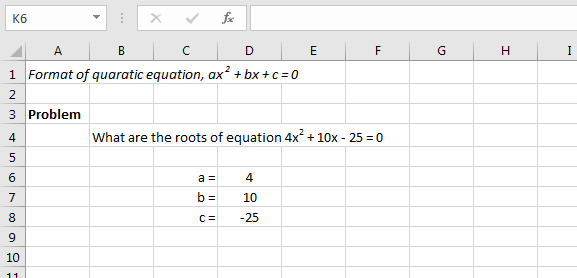Accessing the Visual Basic Window
First step is to go to Developer tab > Visual Basic. In the image below, the visual basic ribbon is at the far left of the menu under developer tab.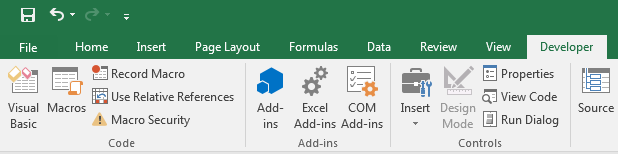Creating an Excel Module
When the VBA window is open, the next step is to right click on Microsoft Excel Objects then Insert > Module. A new blank module will come out where codes can be written.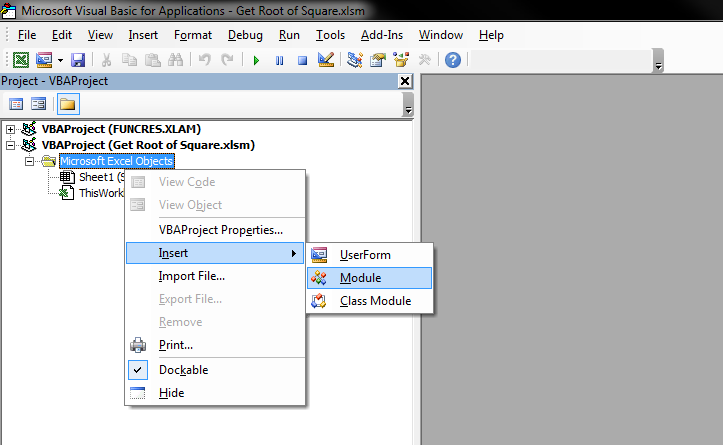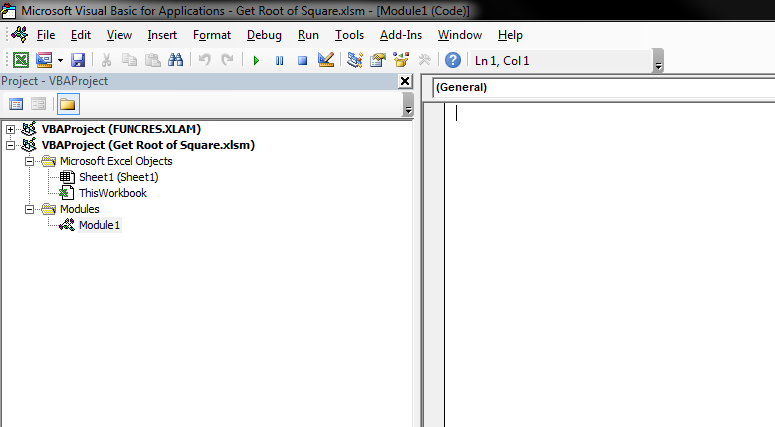Writing the User Function in a VBA Module
Like in a built-in function of Excel, it needs a command name and known variables. The command names in this example are RootXone and RootXtwo; while the known variables are a, b and c. In VBA it is a common practice to define the kind of variables to save memory thus increasing the speed of calculation. The four variables are defined as Double because these can be very large numbers with decimals.
Notice that the way we write formulas in VBA is almost the same in normal Excel sheet. We defined two functions in this case because we have two values of x when getting the roots of quadratic equation. The only difference in the two functions is the + and - expression. Please note that in VBA we are using syntax of Sqr to get the square root while in normal Excel sheet we are using Sqrt.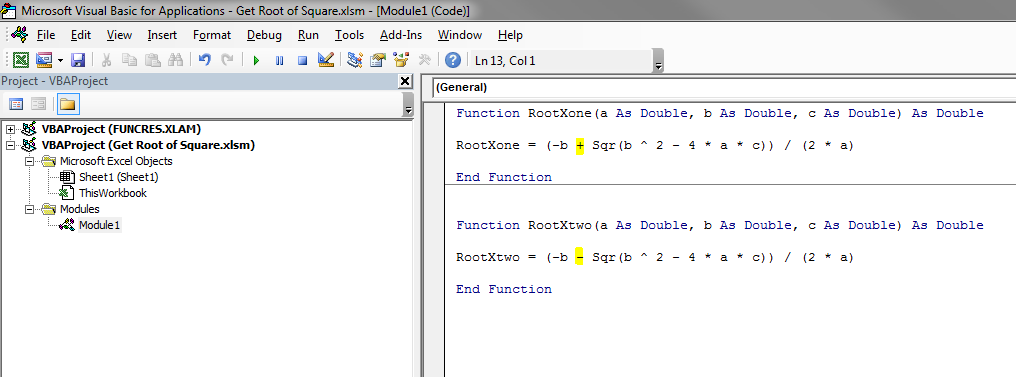Applying the Customized Function in Excel Sheet
To use the user-defined function is the same as using built-in function. First is to call the command then choose the know variables with same arrangement in the VBA module. See below images showing how it is written in the formula bar.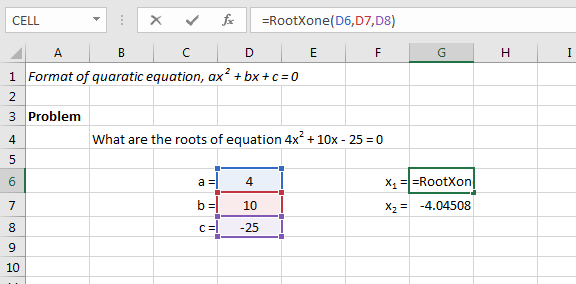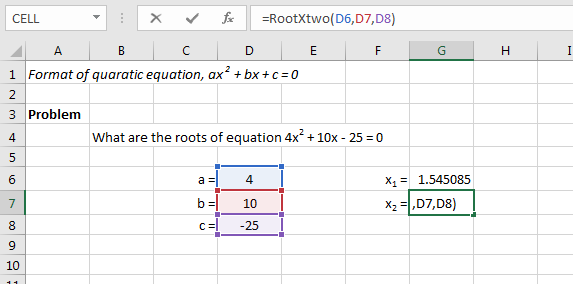Output of the Function
Here it shows the roots of the quadratic equation. The main advantage of a user-defined function is it can be applied in a bunch of data using short commands. It is also very easy to check than combining different built-in functions which will take most of the space in the formula bar.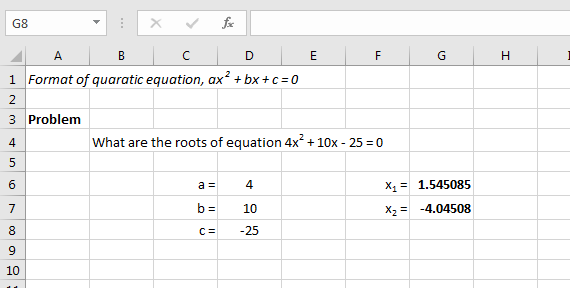More tutorials..
<< Max and If | Count Working Days >>

Excelcrib was founded in November 2017 by Garry, a Microsoft® Office Excel® (MS Excel) enthusiast with background in engineering. He's been using MS Excel for more than 15 years in practice with specialty in VBA.